M340L 3rd Midterm With Solutions, April 7, 2003

1. The following two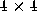matrices are row-equivalent: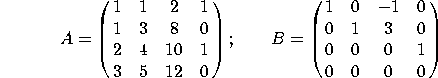a) What is the rank of A?

There are 3 pivots, so the rank is 3.

b) Find a basis for the column space of A.

The pivots are in the 1st, 2nd and 4th columns, so a basis is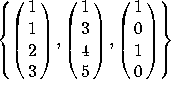. Note that the 1st, 2nd and 4th columns of B are NOT a basis for Col(A). They are a basis for Col(B).

c) Find a basis for the null space of A.

After row-reduction our equations become: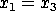,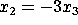,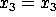, and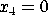, so all solutions are multiples of the single basis vector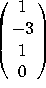.

d) Find a basis for the row space of A.

Since Row(A)=Row(B), our basis is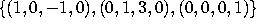. Note that the first three rows of A are NOT a basis, as the third is the sum of the first two.

2. Let V=Span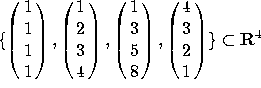.

a) What is the dimension of V?

V is the column space of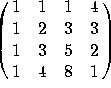, which row-reduces to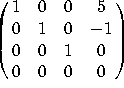. There are 3 pivots, so the dimension is 3.

b) Find a basis for V.

The pivots are in the first 3 columns, so the first three vectors will do, namely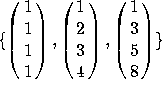c) Let W be the subspace of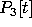spanned by the polynomials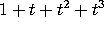,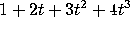,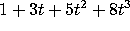and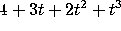. What is the dimension of W? Find a basis for W.

Once you write down the coordinates, this is the EXACT SAME PROBLEM. The dimension is 3, and the basis is the set of vectors whose coordinates are the answer to part (b), namely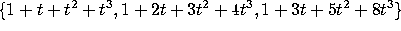.

3. Consider the following basis for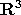: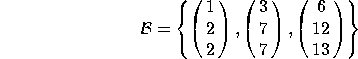a) Find the change-of-basis matrix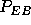that converts from coordinates in the B basis to coordinates in the standard (E) basis.

The columns ofare just the vectors themselves: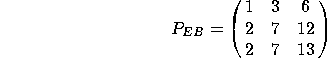b) Find the change-of-basis matrix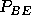that converts from coordinates in the standard basis to coordinates in the B basis.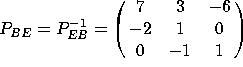, as computed by row-reducing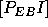.

c) Let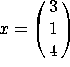. Compute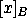.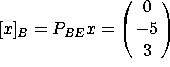. You can check that x is indeed equal to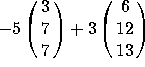.

d) Consider the basis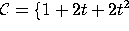,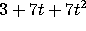,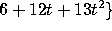for the vector space. Find the coordinates of the polynomial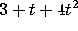in this basis.

Once you write this in coordinates (with respect to the standard basis), this is the same problem as (c), and has the same answer: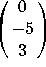4. a) Are the polynomials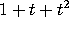,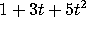and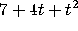linearly independent?

Use coordinates to convert this into a problem in. Row-reducing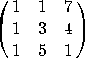gives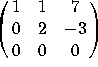, which only has 2 pivots. Since there is not a pivot in the last column, the three vectors are NOT linearly independent.

b) Do the polynomials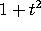,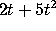and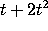span?

Likewise, use coordinates. The matrix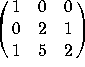DOES row-reduce to something with a pivot in each row, so the vectors DO span the space. (Instead of row-reducing, you could also compute the determinant).

5. Indicate whether each of these statements is true or false. If a statement is sometimes true and sometimes false, write ``false''. You do NOT have to justify your answers. There is no penalty for wrong answers, so go ahead and guess if you are unsure of your answer.

a) The rank of a matrix is the dimension of its row space.

TRUE. It's also the dimension of the column space.

b) Let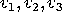be vectors in a vector space V. For Span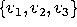to be a subspace of V, the vectors must be linearly independent.

FALSE. If the vectors are linearly dependent, then the dimension of Spanwill be less than 3, but it will still be a subspace.

c) If A and B are row-equivalent matrices, then Col(A)=Col(B).

FALSE. See Problem 1 for a counterexample.

d) If A and B are row-equivalent matrices, then Null(A)=Null(B).

TRUE. The equations Ax=0 and Bx=0 are equivalent.

e) If A and B are row-equivalent matrices, then Row(A)=Row(B).

TRUE. The rows of each can be obtained from the rows of the other.

f) Let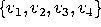be a collection of four vectors in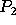. One of the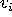's can be written as a linear combination of the others.

TRUE.has dimension 3, so any collection of 4 or more vectors is linearly dependent, so one of the vectors is a linear combination of the others.

g) If a collection of five polynomials spans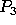, then it forms a basis for.

FALSE.is only 4-dimensional, so any collection of 5 vectors will fail to be linearly independent.

h) Every change-of-basis matrix is invertible.

TRUE.  The inverse of P_{BC} is P_{CB}.

i) If a linearly dependent set of vectors spans, then there must be at least 4 vectors in the set.

TRUE. A linearly dependent set of 3 (or fewer) vectors can't span.

j) If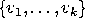is a linearly independent set of vectors in V, and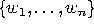is a spanning set for V, then n>k.

FALSE. All you can say is that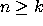.